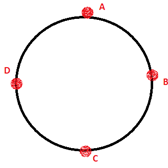Physics
Easy

Question

# As the velocity of the object moving in a uniform circular motion increases, the time period _______.

## IncreasesDecreasesIncreases exponentiallyDecreases exponentiallyHint:

## The correct answer is: Decreases

Physics

Physics

Physics

### A boy moves a tied stone above his head in circles, time period of circular motion is 4.4 sec, and its speed of rotation is 42 m/s. What is the radius of the circle formed?Physics

Physics

Physics

### What is the direction of the velocity at point mentioned if the ball is revolving clockwise.At point DPhysics

Physics

Physics

### What is the direction of the velocity at point mentioned if the ball is revolving clockwise.At point APhysics

Physics

Physics

### An object is moving in a uniform circular motion. It is released while moving in a circular motion. It willPhysics

Physics

Physics

### When is an object said to be accelerated?A student writes the following points: When there is change in speed. When there is change in direction. When there is change in the magnitude of the velocity. Identify the correct option/s.#### With Turito Foundation.#### Get an Expert Advice From Turito.